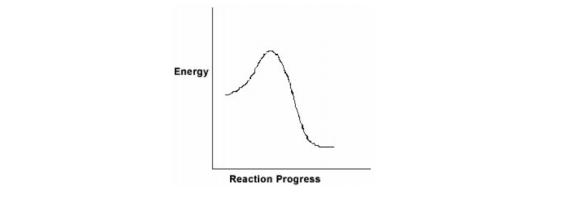# Problem: For the chemical reaction system described by the diagram below, which statement is true  A. The forward reaction is endothermic. B. The activation energy for the forward reaction is greater than the activation energy for the reverse reaction. C. At equilibrium, the activation energy for the forward reaction is equal to the activation energy for the reverse reaction. D. The activation energy for the reverse reaction is greater than the activation energy for the forward reaction. E. The reverse reaction is exothermic.

93% (44 ratings)
###### Problem Details

For the chemical reaction system described by the diagram below, which statement is true

A. The forward reaction is endothermic.

B. The activation energy for the forward reaction is greater than the activation energy for the reverse reaction.

C. At equilibrium, the activation energy for the forward reaction is equal to the activation energy for the reverse reaction.

D. The activation energy for the reverse reaction is greater than the activation energy for the forward reaction.

E. The reverse reaction is exothermic.What scientific concept do you need to know in order to solve this problem?

Our tutors have indicated that to solve this problem you will need to apply the Energy Diagram concept. If you need more Energy Diagram practice, you can also practice Energy Diagram practice problems.

What is the difficulty of this problem?

Our tutors rated the difficulty ofFor the chemical reaction system described by the diagram be...as medium difficulty.

How long does this problem take to solve?

Our expert Chemistry tutor, Jules took 2 minutes and 27 seconds to solve this problem. You can follow their steps in the video explanation above.

What professor is this problem relevant for?

Based on our data, we think this problem is relevant for Professor Krueger's class at UNC.